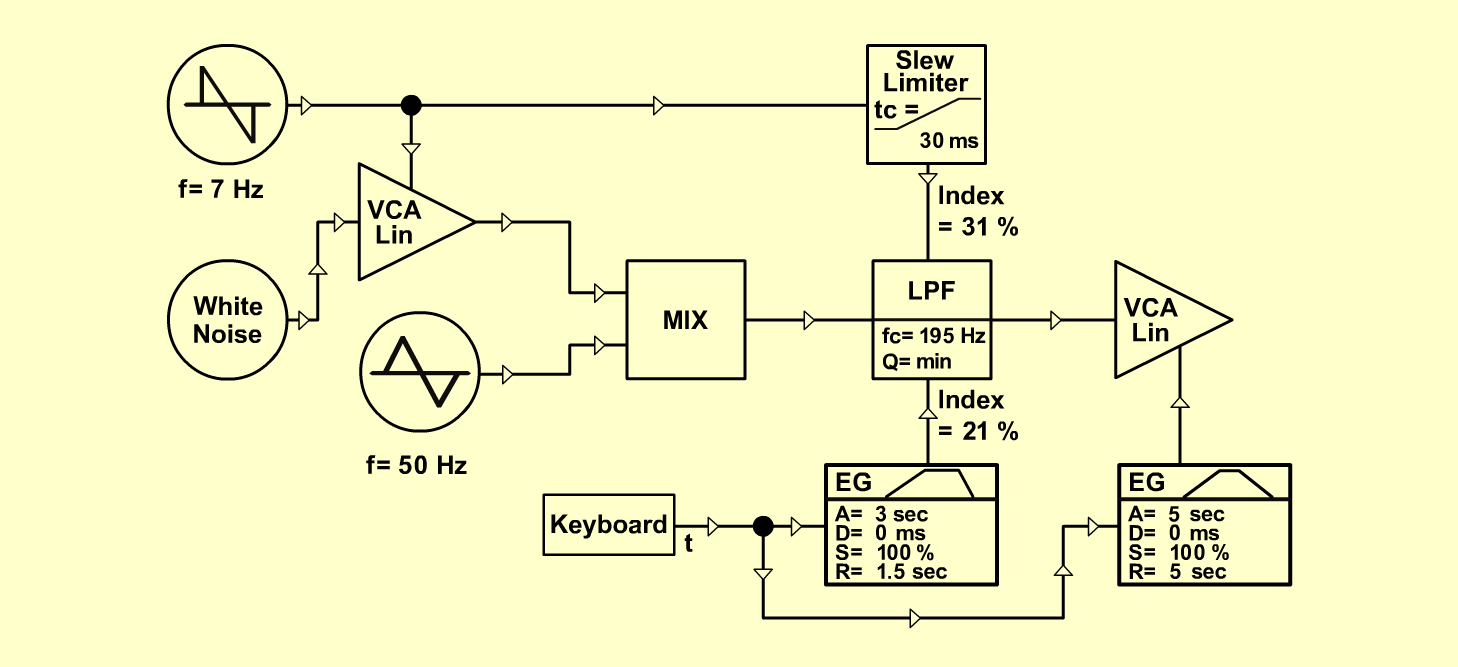ChopperFXD o e p f e r A - 100 Connections: Settings: A-145/1 (Tri)  <=>  A-138/exp (Input 1) A-118 (White)  <=>  A-130 (Audio In) A-130 (Audio Out)  <=>  A-138/exp (Input 2) A-138/exp (Output)  <=>  A-120 (Audio In) A-120 (Audio Out)  <=>  A-131 (Audio In) A-131 (Audio Out)  <=>  Ampifier A-145/2 (Saw down)  <=>  A-180 A-180  <=>  A-130 (CV 1) A-180  <=>  A-170/up (In) A-170 (Out)  <=>  A-120 (CV 3) A-140/1 (Output)  <=>  A-120 (CV 2) A-140/2 (Output)  <=>  A-131 (CV 1) KB Gate  <=>  A-140 (Gate) A-145/1 (Frq = 9, Range = M) A-145/2 (Frq = 3, Range = M) A-138 (In 1 = 10, In 2 = 8.1, Out = 9) A-120 (Freq = 2.9, CV 2 = 2.1, CV 3 = 3.1, Lev = 10, Res = 0) A-131 (Gain = 0, Audio In 1 = 10,  Audio Out = 10) A-140/1 (A = 3, D = 0.2, S = 7, R = 1.5, Range = H) A-140/2 (A = 4, D = 1.5, S = 9.2, R = 3, Range = H) A-170 (Time = 1) A-130 (Gain = 0, Audio In 1 = 10,  Audio Out = 10) Notes: I got the idea from the movie: "Apocalypse now". -The choppers in the beginning sounds something like this one. (I guess they were made by Pat Gleason on his E-mu modular.) Keld Sørensen, November 2001Sound samples Chopper Chopper 2 (with chorus) Chopper 3 (with chorus & pan)